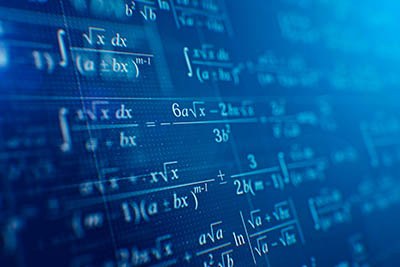# Types of numbers: Difference and classification(© Sashkin– stock.adobe.com)

Numbers comprise a string of digits that are used to represent various quantities and amounts. Each number designates the size of some quantity that is either large or small. Numbers can be of different types and forms, for example, 9, 8/9, 0.599, 56, etc. These numbers can be classified into different subsets based on their properties, such as natural numbers, integers, real numbers, rational and irrational numbers.

## Types of numbers in math

All numbers belong to the same number system, but they have different types. There are various patterns of ten digits(0-9) that are classified into a variety of number types. All these number patterns are separated from each other due to their unique representations and properties.

### Natural numbers

The natural numbers are part of the number system that was historically used. It includes all positive numbers starting from 1,2,3 etc. These numbers do not have zero; zero is considered as a part of the subset of whole numbers. They are also known as positive integers, which are denoted by the symbol N. In the set form; they can be written as: {1, 2, 3, 4, 5, …}

### Whole numbers

Whole numbers are a superset of natural numbers. These numbers include all the positive numbers starting from 1, 2, 3 upto infinity. The whole numbers set also contains zero. All the natural numbers are whole numbers. On the number line, whole numbers are plotted to start from 0, 1, 2, and so on. Some examples of the whole number are 0, 70, 98, 12345, etc. Whole numbers are denoted by the symbol W.

### Integers

Integers are numbers on both sides of zero, which means integers include the complete negative set of natural numbers and whole numbers that have zero and the positive set of natural numbers. Collectively integers are symbolized by the letter Z in the number system. All whole numbers and natural numbers are integers, but not all integers are natural numbers or whole numbers. Few examples of Integers are -10, 5, 0, 23, 100 etc. Integers can be written in a set form as {…, -3, -2, -1, 0, 1, 2, 3, …}

### Fractions

A fraction represents parts of a whole. It is written in the form of a/b, where both a and b are whole numbers and are never equal to 0. Rational numbers include all fraction numbers. Both terminating and repeating decimals can be expressed as fractions. For example, We can write the terminating decimal 0.25 as 25/100 = 5/10 = ½  and a repeating decimal 0.2222 as ½.

### Rational numbers

Rational Numbers are defined as integers and numbers, which can be expressed as a fraction. I.e., a rational number can be written as a fraction of two integers. For example, 0.5 is a rational number because it can be written as 5/10. Other examples of rational numbers are -1/7, 9/5, 77/99, 1.76, etc.

### Irrational numbers

Irrational numbers comprise numbers that cannot be represented in fraction form; that is, irrational numbers cannot be written in a fraction form. Such as the square root of 9 and the value of pi. All irrational numbers are a subset of Real numbers, which implies all irrational numbers are real numbers. The symbol used to represent irrational numbers is Q.

### Real numbers

Real numbers are numbers that represent negative numbers, positive numbers, and zero. The set of real numbers comprises the set of all rational and irrational numbers. Real numbers subset includes integers, fractions, whole numbers, repeating decimals, terminating decimals, etc. The symbol used to represent real numbers is R.

### Imaginary numbers

Numbers that are not a part of the real number subset are called imaginary or complex numbers. For example, √-7 and √-9. The square of these numbers results as -2 and -5. The square root of negative numbers is represented by the letter i, i.e., i = √-1.

Story by Prashant Kumar